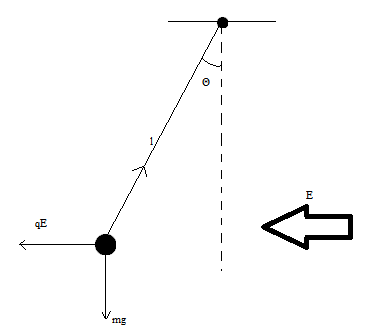# A small plastic ball of mass m = 1.50 g is suspended by a string of length L = 15.5 cm in a...

## Question:

A small plastic ball of mass m = 1.50 g is suspended by a string of length L = 15.5 cm in a uniform electric field. If the ball is in equilibrium when the string makes a 19.0-degree angle with the vertical, what is the net charge on the ball?

## Force balance equations in the presence of electric field:

When there is an electric field, the charged body experiences a force in the direction of the field if it is a positive charge or in a direction opposite to the field if the charge is negative. The field can be simply resolved into the components along the preferred axes and can be taken into account while writing the force balance equation. As in this case, if the field acts at an angle to the vertical/ horizontal, the field can be resolved in its horizontal and vertical components and then introduced into the force balance equation.

The situation here isFor the state of equilibrium, the force balance equations will be

{eq}Tcos\theta=mg\ \ \ \ \ \ \ \text{Along vertical direction}\\ Tsin\theta=qE\ \ \ \ \ \ \ \text{Along horizontal direction}\\ {/eq}

Dividing these equations, we get

{eq}tan\theta=\dfrac{mg}{qE}\\ tan19^\circ=\dfrac{1.5\times10^{-3}\ kg \times 9.8\ m/s^{-2}}{qE}\\ \implies q=\dfrac{1.5\times10^{-3}\ kg \times 9.8\ m/s^{-2}}{tan19^\circ E}\\ \therefore \ q=\dfrac{0.04266}{E} {/eq}

NOTE: Since we are given no information about the electric field, this solution has assumed that the field is in the horizontal direction and the field strength is E. For field in a different direction, the force balance equation will be slightly modified only for the vertical direction. For the horizontal direction, the force balance equation will remain unchanged.